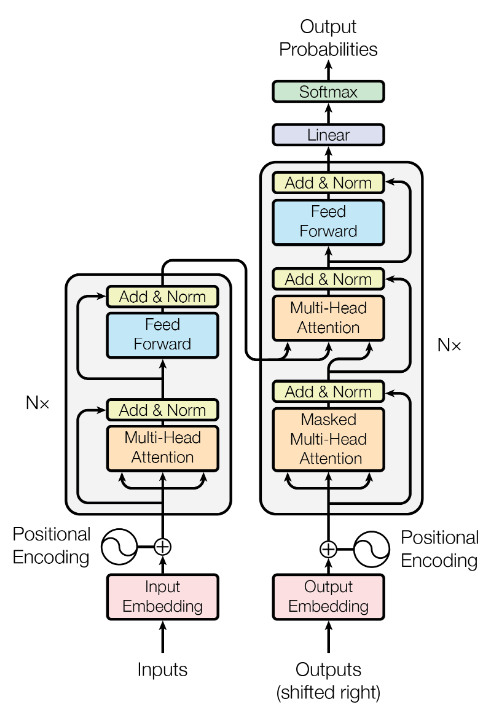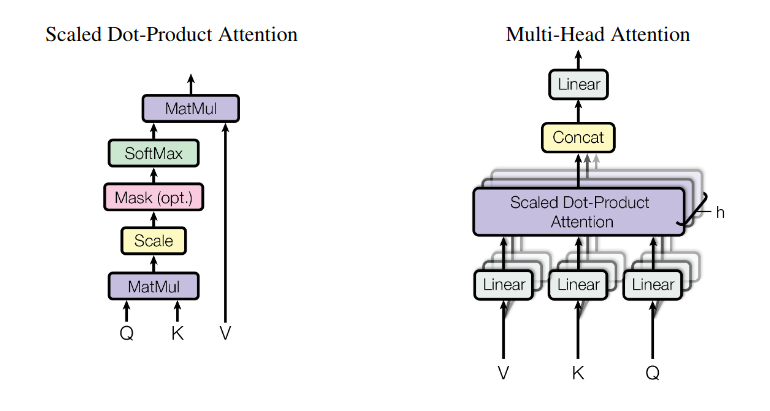# Attention is all you need

Posted on
nlp attention

I also referred to this implementation to understand some of the details.

This is the paper describing the Transformer, a sequence-to-sequence model based entirely on attention. I think it’s best described with pictures.From this picture, I think the following things need explaining:

1. embeddings these are learned embeddings that convert the input and output tokens to vectors of the model dimension. In this paper, they actually used the same weight matrix for input embedding, output embedding, and the final linear layer before the final softmax.
2. positional encoding: since there’s no concept of a hidden state or convolution that encodes the order of the inputs, we have to add some information about the position of the tokens. They used a sinusoidal positional encoding that was a function of the position and the dimension. The wavelength for each dimension forms a geometric progression from $$2\pi$$ to 10000 times that.
3. the outputs are “shifted right”
4. multi-head attention: see below for a description of multi-head attention. In the encoder-decoder attention layers, $$Q$$ comes from the previous masked attention layer and $$K$$ and $$V$$ come from the output of the encoder. Everywhere else uses self-attention, meaning that $$Q$$, $$K$$, and $$V$$ are all the same.
5. masked multi-head attention: in the self-attention layers in the decoder, we can’t allow positions to attend to positions ahead of themselves, so we set all right-connecting values in the input of the softmax (right after scaling; see the image below) to negative infinity.
6. feed-forward blocks these are two linear transformation with ReLU in between. The transformations are the same across each position, but they are different transformations from layer to layer, as you might expect.
7. add & norm: these are residual connections followed by layer normalization.The “Mask (opt.)” can be ignored because that’s for masked attention, described above.

On the left, we can see “dot-product” attention. Additive attention replaces the first matrix multiplication with a feed-forward network with one hidden layer. dot-product attention is faster, so they used it instead. Unfortunately, with large dimension dot-product attention produces large output, so the “scale” block divides its input by the square root of the dimension.

Multi-head attention is just the concatenation of the result of performing attention on several different learned projections of the inputs $$V$$, $$K$$, and $$Q$$. In this paper the projections reduced the dimension such that the resulting multi-head attention had the same overall complexity as regular attention on the unprojected inputs.

Multi-head attention is nice because it allows the output to more easily attend to multiple inputs.

To understand the different dimensions for the tensors used in $$Q$$, $$K$$, and $$V$$ for dot-product attention, check out the implementation here.